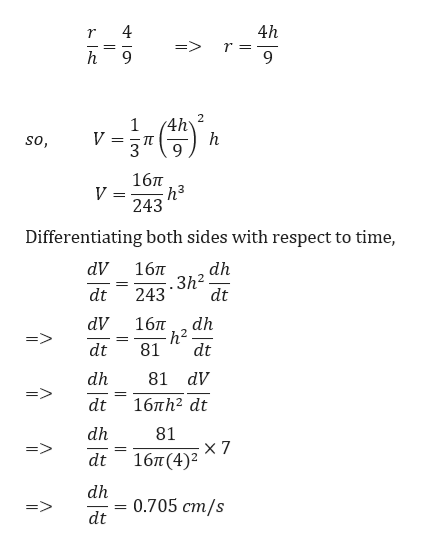# Suppose you are drinking root beer from a conical paper cup. The cup has a diameter of 8 centimeters and a depth of 9 centimeters. As you suck on the straw, root beer leaves the cup at the rate of 7 cm3/sec. At what rate is the level of the root beer in the cup changing when the root beer is 4 centimeters deep?The rate the root beer depth is dropping is (minus)  cm/sec.

Question
4 views

Suppose you are drinking root beer from a conical paper cup. The cup has a diameter of 8 centimeters and a depth of 9 centimeters. As you suck on the straw, root beer leaves the cup at the rate of 7 cm3/sec. At what rate is the level of the root beer in the cup changing when the root beer is 4 centimeters deep?

The rate the root beer depth is dropping is (minus)  cm/sec.

check_circle

Step 1

We need to calculate the rate of change of level of root beer in the conical cup, dh/dt.

We know that, for a cone with base radius r and height h, volume is given by,

Step 2

Given,

Diameter of cup = 8 cm

So, radius of cup = 8/2 = 4 cm

Height of cup = 9 cm

dV/dt =...help_outlineImage Transcriptionclose4h 4 => r= 9 2 1 V 3 h so, 167T h3 V 243 Differentiating both sides with respect to time, dV 167T dh 3h2 243 dt dt dV 16T dh h2 81 dt dt dh 81 dV dt 16Th2 dt dh 81 x 7 16π (4)2 dt dh 0.705 cm/s dt fullscreen

### Want to see the full answer?

See Solution

#### Want to see this answer and more?

Solutions are written by subject experts who are available 24/7. Questions are typically answered within 1 hour.*

See Solution
*Response times may vary by subject and question.
Tagged in

### Derivative